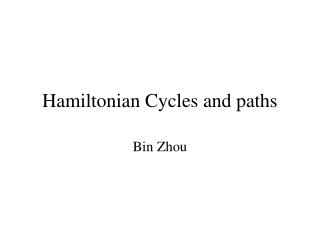# Hamiltonian Cycles and paths - PowerPoint PPT PresentationDownload PresentationHamiltonian Cycles and paths

Hamiltonian Cycles and paths
Download Presentation## Hamiltonian Cycles and paths

- - - - - - - - - - - - - - - - - - - - - - - - - - - E N D - - - - - - - - - - - - - - - - - - - - - - - - - - -
##### Presentation Transcript

1. Hamiltonian Cycles and paths Bin Zhou

2. Definitions • Hamiltonian cycle (HC): is a cycle which passes once and exactly once through every vertex of G (G can be digraph). • Hamiltonian path: is a path which passes once and exactly once through every vertex of G (G can be digraph). • A graph is Hamiltonian iff a Hamiltonian cycle (HC) exists.

3. History • Invented by Sir William Rowan Hamilton in 1859 as a game • Since 1936, some progress have been made • Such as sufficient and necessary conditions be given

4. History • G.A. Dirac, 1952, If G is a simple graph with n(>=3) vertices, and if the degree of each is at least 1/2n, then G is Hamiltonian • O.Ore , 1960 If G is a simple graph with n(>=3) vertices, and if the sum of the degrees of each pair of non-adjacent vertices is at least n, then G is Hamiltonian

5. History • Bondy and Chvatal , 1976 For G to be Hamiltonian, it is necessary and sufficient that [G]n be Hamiltonian. ([G]n is gotten from G by adding edges joining non-adjacent vertices whose sum of degrees is equal to, or greater than n)

6. History • Fraudee, Dould, Jacobsen, Schelp (1989) If G is a 2-connected graph such that for every pair of nonadjacent nodes u and v, |d(u)+d(v)|>=(2n-1)/3 then G is Hamiltonian

7. Application • Hamiltonian cycles in fault random geometric network • In a network, if Hamiltonian cycles exist, the fault tolerance is better.

8. Hamiltonian problem is NPC • This is a well known NP complete problem • For general graph, we can not find an exactly linear time complexity algorithm to find a Hamiltonian cycle or path

9. HC algorithms • For general graphs, no efficient algorithm NP-complete for perfect graphs, planar bipartite graphs, grid graphs, 3-connected planar graphs • For some special graphs, exist efficient algorithms. N. Ghiba, T. Nishizeki (1989) Polynomial algorithm for 4-connected planar graphs. G.Gutin (1997) Polynomial algorithm for quasi-transitive digraphs

10. Some Algorithms for HC • L. Pósa (1976) Rotational transformation • B. Bollobás, T.I.Fenner, and A. M. Frieze Cycle extension (HAM) (1987) • Silvano Martello Algorithm 595 (1983)

11. Two classes of algorithms • Heuristic algorithm Pósa, UHC, DHC, HAM, etc • Backtrack algorithm 595HAM, KTC, MultiPath

12. Backtrack Algorithm • Recurse(Path p, endpoint e) • While (e has unvisited neighbors) { GetNewNode x; (add x node to P) PruneGraph. (Prune graph. If result graph does not permit a HC forming, remove x from P and continue) FormCycle (If P includes all nodes then try to form cycle. Fail, remove x and continue; Succ, return success) BackTrack: Recurse(P,x) } Return fail.

13. Backtrack Algorithm • Search all the potential solutions • Employ pruning of some kind to restrict the amount of researching • Advantage: Find all solution, can decide HC exists or not • Disadvantage Worst case, needs exponential time. Normally, take a long time

14. Heuristic Algorithm Initialize path P While { Find new unvisited node. If found { Extend path P and pruning on the graph. If this choice does not permit HC, remove the extended node. } else Transform Path. Try all possible endpoints of this path Form cycle. Try to find HC }

15. Heuristic Algorithm • Advantage: Fast. Linear or low-order polynomial time • Disadvantage Maybe can not find the HC

16. Ham heuristic algorithm • Try to extend existing path and never decrease the path length • Do cycle extension • Do rotational transformation

17. Ham algorithm Start from a random node and find a neighbor to get a path P. |P|=2 Do { Change partial path array A. oldlength=|P|. While |P|==oldlength { Find neighbors of P’s endpoints.Try to extend P. For (each neighbor) do { If Extendable Extend and continue; Else Do cycle extension or rotational transformation; } Check termination condition and change P } }}

18. Cycle Extention Path P: x1 xi xi+1 xk u Path P’: u xi x1 xk xi+1

19. Rotational transformation Path P: x1 xi xi+1 xk Path P’: xi x1 xi+1 xk

20. Results

21. Results

22. Results

23. Problems • The program can not check large graph due to the memory restriction • May be need more conditions to decide the probability of HC exists • We can solve large problem using parallel computing